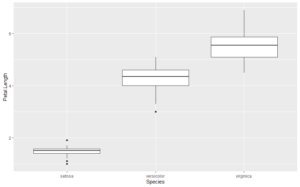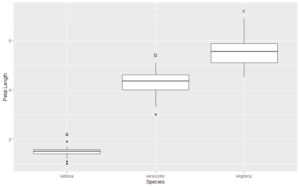# Compact Letter Displays

Compact letter displays (CLDs) are letters that show which treatment groups are not significantly different by some statistical test. It is often desirable to include CLDs on graphs. Here I show how to add them to a box plot created with ggplot2.

First, make an example plot using the iris data:

```library(ggplot2)
library(car)
library(QsRutils)
data("iris")
plt1 <- ggplot(data = iris, aes(x=Species, y=Petal.Length)) + geom_boxplot()
plt1```I want to add CLDs just above the tops of the box whiskers in the plot. I can use the base boxplot function to capture the coordinates for these points.

```box.rslt <- with(iris, graphics::boxplot(Petal.Length ~ Species, plot = FALSE))
str(box.rslt)
List of 6
\$ stats: num [1:5, 1:3] 1.1 1.4 1.5 1.6 1.9 3.3 4 4.35 4.6 5.1 ...
\$ n    : num [1:3] 50 50 50
\$ conf : num [1:2, 1:3] 1.46 1.54 4.22 4.48 5.37 ...
\$ out  : num [1:2] 1 3
\$ group: num [1:2] 1 2
\$ names: chr [1:3] "setosa" "versicolor" "virginica"```

The fifth row of `box.rslt\$stats` gives the y coordinates for the tops of the whiskers.

```box.rslt\$stats
[,1] [,2] [,3]
[1,] 1.1 3.30 4.50
[2,] 1.4 4.00 5.10
[3,] 1.5 4.35 5.55
[4,] 1.6 4.60 5.90
[5,] 1.9 5.10 6.90```

Next I have to get a vector of the letters to add to the plot. For this example, I will make a pairwise t-test which outputs a matrix of p-values.

`ptt.rslt <- with(iris, pairwise.t.test(Petal.Length, Species, pool.sd = FALSE))`

Looking at the structure of `ptt.rslt`, we see that `ptt.rslt\$p.value` gives a matrix of p.values:

```str(ptt.rslt)

List of 4
\$ method : chr "t tests with non-pooled SD"
\$ data.name : chr "Petal.Length and Species"
\$ p.value : num [1:2, 1:2] 1.99e-45 2.78e-49 NA 4.90e-22
..- attr(*, "dimnames")=List of 2
.. ..\$ : chr [1:2] "versicolor" "virginica"
.. ..\$ : chr [1:2] "setosa" "versicolor"
- attr(*, "class")= chr "pairwise.htest"

ptt.rslt\$p.value

setosa      versicolor
versicolor 1.986887e-45 NA
virginica 2.780888e-49 4.900288e-22```

From this matrix we can use `QsRutils::make_letter_assignments` to get a vector of letters for our CLDs.

```ltrs <- make_letter_assignments(ptt.rslt)
str(ltrs)

List of 3
\$ Letters          : Named chr [1:3] "a" "b" "c"
..- attr(*, "names")= chr [1:3] "setosa" "versicolor" "virginica"
\$ monospacedLetters: Named chr [1:3] "a  " " b " "  c"
..- attr(*, "names")= chr [1:3] "setosa" "versicolor" "virginica"
\$ LetterMatrix     : logi [1:3, 1:3] TRUE FALSE FALSE FALSE TRUE FALSE ...
..- attr(*, "dimnames")=List of 2
.. ..\$ : chr [1:3] "setosa" "versicolor" "virginica"
.. ..\$ : chr [1:3] "a" "b" "c"
- attr(*, "class")= chr "multcompLetters"

ltrs\$Letters
setosa versicolor  virginica
"a"        "b"        "c"```

`ltrs\$Letters` gives the vector of letters that we want. Now we can make a data frame to add the CLDs to the box plot.

```x <- c(1:length(ltrs\$Letters))
y <- box.rslt\$stats[5, ]
cbd <- ltrs\$Letters
ltr_df <- data.frame(x, y, cbd)
ltr_df
x   y cbd
setosa     1 1.9   a
versicolor 2 5.1   b
virginica  3 6.9   c

```

If we plot the CLDs at the coordinates in `ltr_df`, they will over plot the tops of the whiskers . We need to nudge the CLDs upward to avoid the overlap. To determine how much to nudge, I will get the range of the Y-axis and nudge upward 5% of this range.

```lmts <- get_plot_limits(plt1)
y.range <- lmts\$ymax - lmts\$ymin
y.nudge <- 0.05 * y.range
plt1 +
geom_text(data = ltr_df, aes(x=x, y=y, label=cbd), nudge_y = y.nudge)

```The CLDs are perfectly positioned, and without any trial and error.